# Security Analysis and Portfolio Management – Financial Management MCQ

Security Analysis and Portfolio Management – CS Executive Financial and Strategic Management MCQ Questions with Answers you can quickly revise the concepts.

## Security Analysis and Portfolio Management – Financial Management MCQ

Question 1.
Security Analysis is a process of estimating for individual securities.
(A) Return and risk
(B) Risk and correlation
(C) Correlation and coefficient
(D) Return and coefficient
(A) Return and risk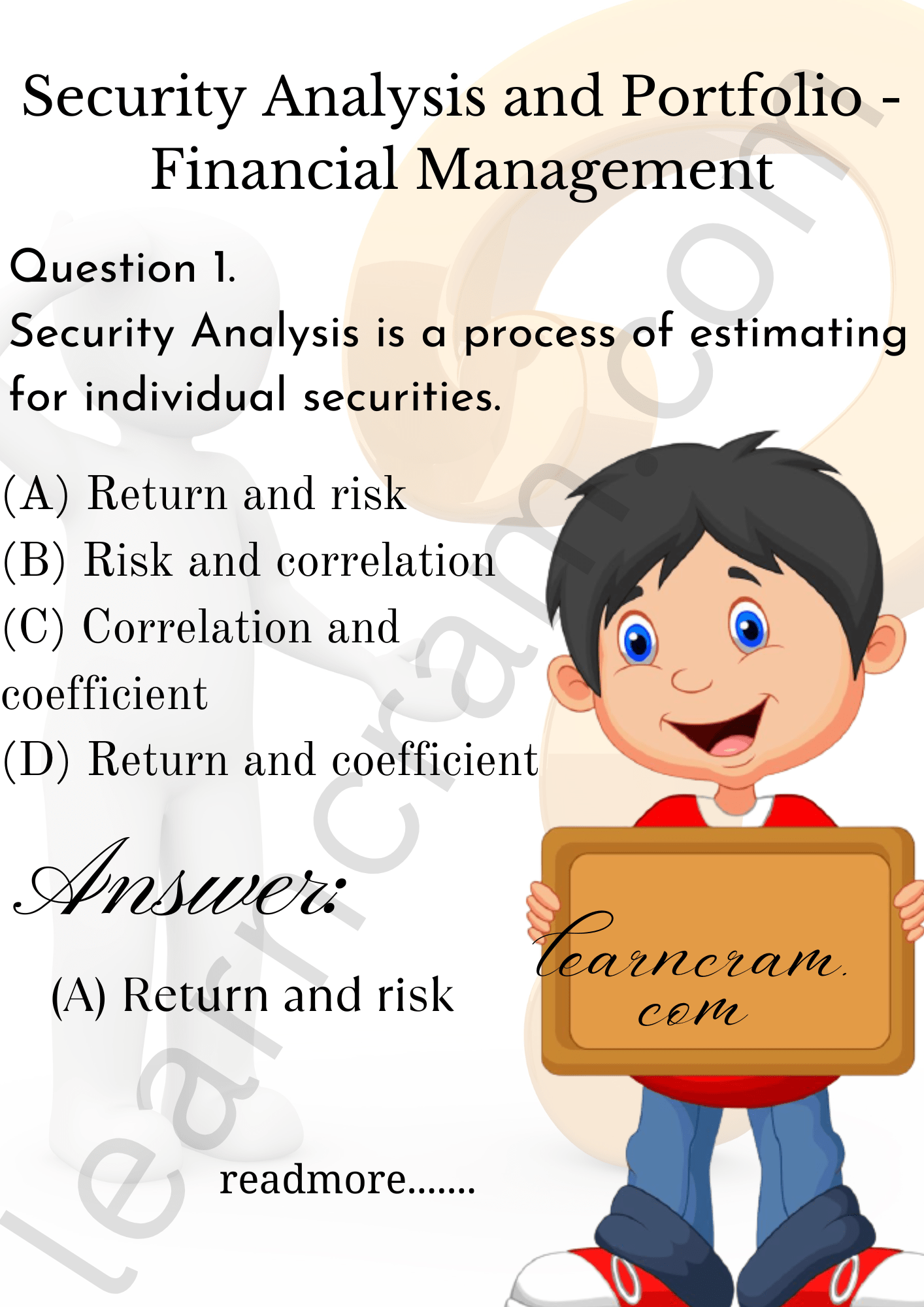Question 2.
Standard deviation determine -…………..
(A) Systematic risk of a security
(B) Unsystematic risk of security
(C) Total risk of security
(C) Total risk of security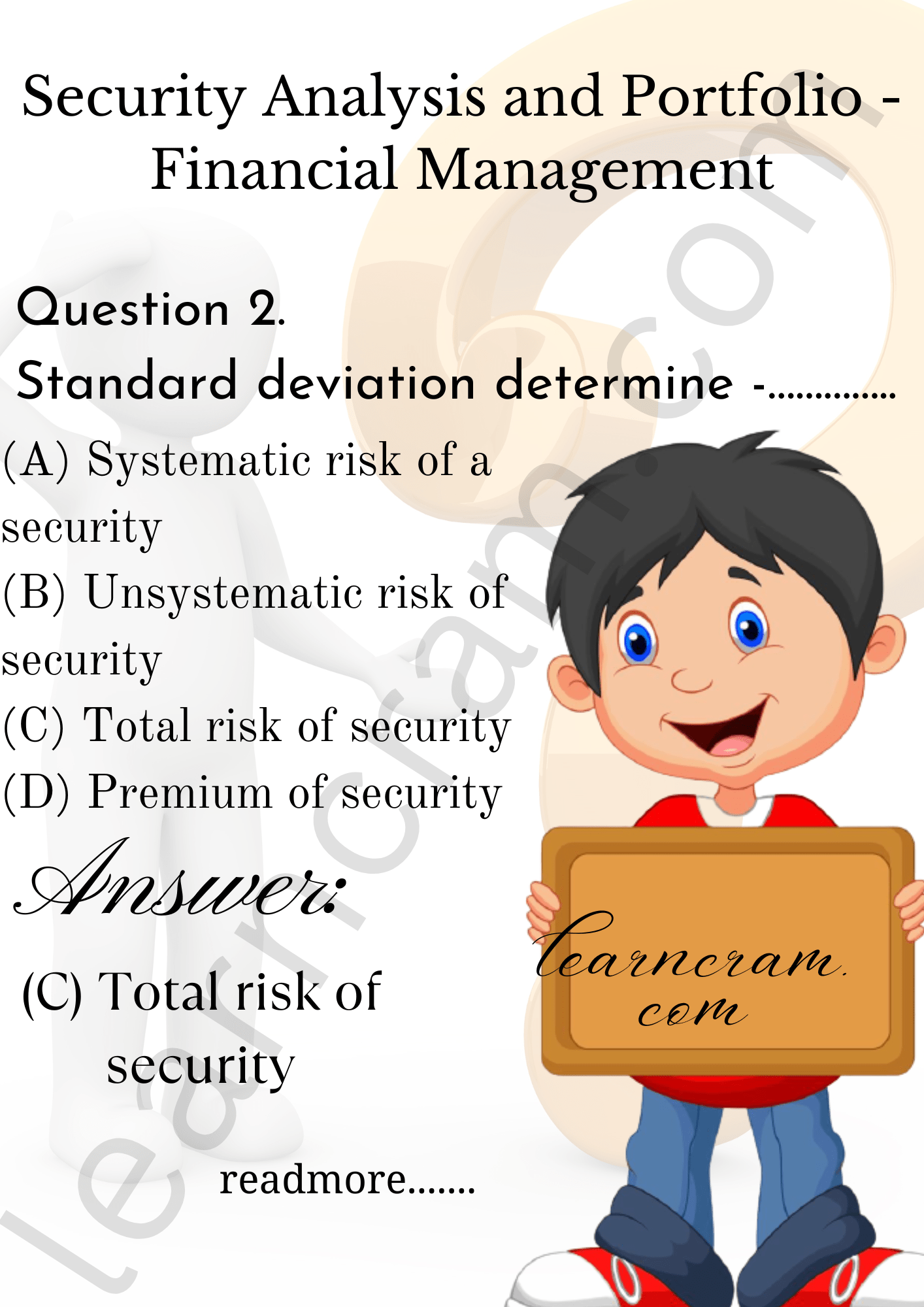Question 3.
Financial Assets are -…………
(A) Pieces of paper representing an indirect claim to real assets in form of debt or equity commitments.
(B) Tangible, material things such as buildings, furniture, automobiles etc.
(C) Both (A) and (B)
(D) Neither (A) nor (B)
(A) Pieces of paper representing an indirect claim to real assets in form of debt or equity commitments.

Question 4.
………….. is one who exercises any degree of discretion as to the investment or management of the portfolio of the securities or the funds of the client.
(A) Non-discretionary portfolio manager
(B) Portfolio investor
(C) Discretionary portfolio manager
(D) Portfolio custodian
(C) Discretionary portfolio manager

Question 5.
Return from listed security is in two forms –
(A) One is interest and second is capital appreciation in price.
(B) One is stock split and second is dividend.
(C) One is interest and second is dividend.
(D) One is dividend and second is capital appreciation in price.
(D) One is dividend and second is capital appreciation in price.

Question 6.
Which of the following is correct formula to calculate returns of listed security?
(A) [(P1 – P0) + D] ÷ [P0 x 100]
(B) [(P1 – P0) + D] ÷ [P0 x 100]
(C) [(P1 – P0) – D] ÷ [P0 x 100]
(D) [(P1 – P0) + D (1 -t)] ÷ [P0 x 100]
(A) [(P1 – P0) + D] ÷ [P0 x 100]

Question 7.
If probability of occurrence is assigned, then the expected return would be:
(A) average return being assigned to return of security for the various scenarios
(B) weighted average of probabilities being assigned to return of security for the various scenarios
(C) weighted average return with probabilities being assigned to return of security for the various scenarios
(D) weighted average return multiplied by risk with probabilities being assigned to return of security for the various scenarios
(C) weighted average return with probabilities being assigned to return of security for the various scenarios

Question 8.
Standard deviation is a deviation from -……………
(A) Arithmetic mean
(B) Harmonic mean
(C) Median mean
(D) Mode mean
(A) Arithmetic mean

Question 9.
Standard deviation is expressed -……………
(A) always in percentage
(B) in same units in respect of which the deviation is computed.
(C) in terms of rupee risk
(D) in terms of amount
(B) in same units in respect of which the deviation is computed.

Question 10.
The market price of a bond depend on -……………
(A) The coupon rate and terms of the indenture
(B) The coupon rate and maturity date
(C) The terms of the indenture, and maturity date
(D) The coupon rate, terms of the indenture, and maturity date
(D) The coupon rate, terms of the indenture, and maturity date

Question 11.
Investment with lower standard deviation carries -……………
(A) High risk
(B) Less risk
(C) Infinite risk
(D) Avoidable risk
(B) Less risk

Question 12.
Which of the following is on the horizontal axis of the Security Market Line?
(A) Standard deviation
(B) Beta
(C) Expected return
(D) Required return
(B) Beta

Question 13.
Covariance is a measurement of -……………
(A) The co-movement between two variables
(B) The link between the variability of returns in two independent securities
(C) Both (A) and (B)
(D) None of the above
(C) Both (A) and (B)

Question 14.
Expected worth is the –
(A) Inverse of standard deviation
(B) Correlation between a security
(C) Same as discrete probability distribution
(D) Weighted average of all possible outcomes
(D) Weighted average of all possible outcomes

Question 15.
Positive Covariance indicates that –
(A) Returns on two assets bear a tendency to off-set each other Le. if return on A is above par, return on B is likely to be below par. If return on A is below par, return on B is likely to be above par.
(B) There is no distinct relationship between the movements in returns of two securities.
(C) Returns on two assets tend to go together, ie. if return on A is above par, return on B is also likely to be above par.
(D) Higher discount rate should be used in capital budgeting to discount the cash flow.
(C) Returns on two assets tend to go together, ie. if return on A is above par, return on B is also likely to be above par.

Question 16.
Liquidity risk:
(A) is risk investments bankers face
(B) is lower for small companies
(C) is risk associated with secondary market transactions
(D) increases whenever interest rates increases
(C) is risk associated with secondary market transactions

Question 17.
Correlation Coefficient supplements and upgrades the –
(A) Expected return
(B) WACC
(C) Covariance
(D) Mean deviation
(C) Covariance

Question 18.
Consider a graph with standard deviation on the horizontal axis and expected return on the vertical axis. The line that connects the risk-free rate and the optimal risky portfolio is called:
(A) Indifference curve
(B) Capital market line
(C) Characteristic line
(D) Security market line
(B) Capital market line

Question 19.
A risk associated with project and way considered by well diversified stockholder is classified as –
(A) Expected risk
(B) Beta risk
(C) Industry risk
(D) Returning risk
(B) Beta risk

Question 20.
Which of the following is correct formula to Correlation Coefficient?
(A) Covxy x σ x x σy
(B) Covxy÷ (σx × σy)
(C) (σx x σy) ÷ Covxy
(D) (σx ÷ σy) x Covxy
(B) Covxy÷ (σx × σy)

Question 21.
An attempt to make correction by adjusting historical beta to make it closer to Em average beta is classified as –

Question 22.
The efficient frontier –
(A) Is the set of optimal portfolios that offers the highest expected return for a defined level of risk’
(B) Is the set of optimal portfolios that offers the lowest risk for a given level of expected return
(C) Helps to decide whether a new security can be selected or rejected
(D) All of the above
(D) All of the above

Question 23.
A corporate bond is a corporation’s write undertaking that it will refund a specific amount of money plus –
(B) Interest
(C) Nothing
(D) Security
(B) Interest

Question 24.
The common stock of a company must provide a higher expected return than the debt of the same company because
(A) There is less demand for stock than for bonds.
(B) There is greater demand for stock than for bonds.
(C) There is more systematic risk involved for the common stock.
(D) There is a market premium required for bonds.
(C) There is more systematic risk involved for the common stock.

Question 25.
As per ‘Efficient Frontier’ concept, if the security falls below the frontier –
(A) The security is efficient and hence will be selected.
(B) The security dominates some security on previously drawn security and hence the frontier itself will have to be re-draw.
(C) The security is dominated by some security on the frontier and will be rejected.
(D) It shows that earning of the security is above market return and hence will be selected.
(C) The security is dominated by some security on the frontier and will be rejected.

Question 26.
Capital Market Line is firstly initiated by:
(A) Mohsin
(B) Linter
(C) Markowitz
(D) William Sharpe
(D) William Sharpe

Question 27.
For an all-equity financed firm, a project whose expected rate of return plots should be rejected.
(A) Above the characteristic line
(B) Above the security market line
(C) Below the security market line
(D) Below the characteristic line
(C) Below the security market line

Question 28.
Portfolio risk will be when two components of a portfolio stand perfectly positively correlated and will be when the same are perfectly negatively correlated.
(A) Minimum; Maximum
(B) Maximum; Minimum
(C) Minimum; Less
(D) Maximum; More
(B) Maximum; Minimum

Question 29.
A main difference among real and nominal interest proceeds is that -……….
(A) Real returns adjust for inflation and nominal returns do not
(B) Real returns use actual cash flows and nominal use expected cash flows
(C) Real interest adjusts for commissions and nominal returns do not
(D) Real returns show highest possible return and nominal returns show lowest possible return
(A) Real returns adjust for inflation and nominal returns do not

Question 30.
The beta of the market is: -…………
(A) -1.0
(B) 0
(C) 1.0
(D) 0.5
(C) 1.0

Question 31.
Non-systematic risk is furthermore identified as -…………
(A) No diversiable risk
(B) Market risk
(C) Random risk
(D) Company specific risk
(D) Company specific risk

Question 32.
Beta is measure of -…………
(A) Non-diversifiable risk
(B) Diversihable risk
(C) Total risk
(D) Covariance
(A) Non-diversifiable risk

Question 33.
Investors should be agreeing to invest in riskier investments merely –
(A) If return is short
(B) If there are no safe alternatives except for holding cash
(C) If expected return is adequate for risk level
(D) If there are true speculators
(C) If expected return is adequate for risk level

Question 34.
Negative covariance indicates that -………..
(A) Returns on two assets bear a tendency to off-set each other i.e. if return on A is above par, return on B is likely to be below par. If return on A is below par, return on B is likely to be above par.
(B) Returns on two assets tend to go together, ie. if return on A is above par, return on B is also likely to be above par.
(C) There is no distinct relationship between the movements in returns of two securities.
(D) There is unnecessary relationship between the movements in returns of two securities.
(A) Returns on two assets bear a tendency to off-set each other i.e. if return on A is above par, return on B is likely to be below par. If return on A is below par, return on B is likely to be above par.

Question 35.
Holding two securities as an alternative of will not decrease hazard occupied by an investor if two securities are:
(A) Perfectively positive correlated
(B) Perfectively negative correlated
(C) No correlation
(D) All of answers are correct
(A) Perfectively positive correlated

Question 36.
A beta of 1.15 for a security would indicate that -………….
(A) Security is trading 15% higher than market index
(B) Security is 15% riskier than index/ market.
(C) Security is 15% less risky than index/ market.
(D) Security and market has covariance of 0.15.
(B) Security is 15% riskier than index/ market.

Question 37.
Choice of correlation coefficient is between -………….
(A) 0 to 1
(B) 0 to 2
(C) Minus 1 to +1
(D) Minus 1 to 3
(C) Minus 1 to +1

Question 38.
A beta of 0.8 for a security would indicate that -………….
(A) Security is 20% riskier than index/ market.
(B) Security is 80% less risky than index/ market.
(C) Security is 20% less risky than index/ market.
(D) Security is 80% riskier than index/ market
(C) Security is 20% less risky than index/ market.

Question 39.
Markowitz model presumed generally investors are –
(A) Risk averse
(B) Risk natural
(C) Risk seekers
(D) Risk moderate
(A) Risk averse

Question 40.
Zero covariance indicate that –
(A) There is close relationship between the movements in returns of two assets.
(B) There is positive relationship between the movements in returns of two securities.
(C) There is strong relationship between the movements in returns of two assets.
(D) There is no distinct relationship between the movements in returns of two securities.
(D) There is no distinct relationship between the movements in returns of two securities.

Question 41.
Which of the following is correct formula to calculate beta (P)?(D) All of the Above

Question 42.
Capital Asset Pricing Model (CAPM) provides the link between –
(A) Return and total risk
(B) Risk and covariance
(C) Return and non-diversifiable risk
(D) Return and diversifiable risk
(C) Return and non-diversifiable risk

Question 43.
Which of the following investment advice will you provide to your client investor if CAPM Return < Expected return?
(A) Sell
(B) Hold
(D) Short

Question 44.
As per ‘Efficient Frontier’ concept, if the security falls on frontier –
(A) The security is efficient and hence will be selected
(B) The security dominates some security on previously drawn security and hence the frontier itself will have to be re-drawn
(C) The security is efficient but will be rejected.
(D) The security is dominated by some security on the frontier and will be rejected.
(A) The security is efficient and hence will be selected

Question 45.
Capital market line (CML) represents –
I. Portfolios that optimally combine risk and return.
II. The trade-off between risk and return for efficient portfolios.
III. Covariance value in order to help comparison with corresponding values for the other pairs of securities constituting the portfolio.
IV. The relationship between return and risk.
Select the correct answer from the options given below.
(A) IV and I only
(B) II, III and IV only
(C) III and n only
(D) III, IV and I only
(D) III, IV and I only

Question 46.
Which of the following investment advice will you provide to your client investor if CAPM Return > Expected return?
(A) Sell
(C) Hold
(D) None of the above
(A) Sell

Question 47.
If expected return is more than required return as per CAPM, then -…………
(A) Security is overvalued and hence can be bought
(B) Security is correctly priced and hence should be hold
(C) Security is undervalued and hence can be sold
(D) Security is undervalued and hence can be bought
(C) Security is undervalued and hence can be sold

Question 48.
The Security Market Line (SML) is a line drawn on a chart that serves as a graphical representation of the -…………
(A) MM Model
(B) Capital Asset Pricing Model
(C) Markowitz Model
(D) NOI Model
(C) Markowitz Model

Question 49.
The opportunity line is the:
(A) Set of all portfolios with the same expected rate of return but different standard deviations.
(B) Set of investment opportunities made available by mixing a risky asset and a risk-free asset.
(C) Set of investment opportunities made available by mixing two risky assets.
(D) Set of portfolios among which the investor is indifferent.
(B) Set of investment opportunities made available by mixing a risky asset and a risk-free asset.

Question 50.
The Security Market Line (SML) is a line drawn on a chart that serves as a graphical representation of the Capital Asset Pricing Model, which shows different levels of …………. of various marketable securities
plotted against the expected return.
(A) Systematic risk
(B) Statement Q is true and Statement P is false.
(C) Both Statement P and Statement Q are true.
(D) Both Statement P and Statement Q are false.
(A) Systematic risk

Question 51.
Market risk is also called: ………
(A) Systematic risk and unique risk.
(B) Unique risk & non diversifiable risk.
(C) Systematic risk & diversihable risk.
(D) Non diversifiable risk & systematic risk.
(D) Non diversifiable risk & systematic risk.

Question 52.
As per ‘Efficient Frontier’ concept, if the security falls above the frontier –
(A) the security is dominated by some security on previously drawn security and hence the frontier itself will have to be re-drawn.
(B) the security dominates some security on previously drawn security and hence the frontier itself will have to be re-drawn.
(C) the security is dominated by some security on the frontier and will be rejected.
(D) the security is efficient and hence will be selected.
(B) the security dominates some security on previously drawn security and hence the frontier itself will have to be re-drawn.

Question 53.
Which of the following investment advice will you provide to your client investor if CAPM Return = Expected return?
(A) Sell
(C) Hold
(D) Put
(C) Hold

Question 54.
If required return as per CAPM is more than expected return, then –
(A) Security is undervalued ……….. and can be sold
(B) Security is correctly priced and hence should be hold
(C) Security is overvalued and hence can be bought
(D) Security is undervalued and hence can be bought
(A) Security is undervalued ……….. and can be sold

Question 55.
If an asset’s expected return plots above the security market line, the asset is:
(A) Overpriced
(B) Underpriced
(C) Fairly priced (if it has an unusually large amount of unique risk)
(D) Both the first and third answers.
(B) Underpriced

Question 56.
The X-axis of the Security Market Line (SML) chart represents –
(A) Expected return
(B) Risk in terms of beta
(C) Standard deviation
(D) Co-efficient
(B) Risk in terms of beta

Question 57.
The market risk premium is the slope of the:
(A) Security market line
(B) Opportunity line
(C) Efficient frontier
(D) Capital market line
(A) Security market line

Question 58.
…………. is also called a Characteristic Line.
(A) Capital Market Line
(B) Security Market Line
(C) Opportunity line
(D) Line of expected return
(B) Security Market Line

Question 59.
According to the CAPM, overpriced securities have:
(A) Negative alphas
(B) Positive alphas
(C) Zero betas
(D) Zero alphas
(A) Negative alphas

Question 60.
Consider following two statements:
P. Beta coefficient is the measure of risk in CML.
Q. Standard deviation determines the risk factors of the SML.
Select correct answer from the options given below.
(A) Statement P is true and Statement Q is false.
(B) Statement Q is true and Statement P is false.
(C) Both Statement P and Statement Q are true.
(D) Both Statement P and Statement Q are false.
(D) Both Statement P and Statement Q are false.

Question 61.
The beta of the risk-free asset is:
(A) 0
(B) 0.5
(C) 2.0
(D) 1.0
(A) 0

Question 62.
Security Market Line graphs defines -………
(A) Efficient portfolios
(B) Non-efficient portfolios
(C) Disposable portfolios
(D) Both efficient and non-efficient portfolios.
(D) Both efficient and non-efficient portfolios.

Question 63.
Capital asset pricing theory asserts that portfolio returns are best explained by:
(A) Economic factors
(B) Systematic risk
(C) Specific risk
(D) Diversification
(B) Systematic risk

Question 64.
According to security market line, the expected return of any security is a function of:
(A) Diversifiable risk
(B) Unique risk
(C) Unsystematic risk
(D) Systematic risk
(D) Systematic risk

Question 65.
According to the capital market line, the expected return of any efficient portfolio is a function of:
(A) Unsystematic risk
(B) Systematic risk
(C) Unique risk
(D) Total risk
(D) Total risk

Question 66.
Which of the following statements about the market portfolio is false?
(A) The market portfolio is on the efficient frontier.
(B) The market portfolio lies, on the security market line.
(C) The market portfolio contains both systematic and unsystematic risk.
(D) The market portfolio lies on the capital market line.
(C) The market portfolio contains both systematic and unsystematic risk.

Question 67.
Alpha is an indicator of the extent to which the –
(A) Actual return of a security deviates from those predicated by market experts.
(B) Actual return of a security deviates from those predicated by derivative market
(C) Actual return of a security deviates from those predicated by its beta value.
(D) Actual return of a security deviates from those predicated by its probable distribution value.
(C) Actual return of a security deviates from those predicated by its beta value.

Question 68.
Alpha is denoted by symbol -………
(A) A
(B) ¥
(C) α
(D) Δ
(C) α

Question 69.
Negative alpha value indicates that -………
(A) Expected return is less than required return as per CAPM and hence security is overvalued. Such security should be sold.
(B) Expected return is less than required return as per CAPM and hence security is undervalued. Such security should be bought.
(C) Expected return is more than required return as per CAPM and hence security is undervalued. Such security should be bought.
(D) Expected return is equal to required return as per CAPM and hence security is correctly valued. Such security should be hold.
(A) Expected return is less than required return as per CAPM and hence security is overvalued. Such security should be sold.

Question 70.
Systematic Risk is -………
(A) Uncontrollable
(B) Controllable
(C) Avoidable
(D) Voidable
(A) Uncontrollable

Question 71.
Positive alpha value indicates that –
(A) Expected return is less than required return as per CAPM and hence security is overvalued. Such security should be sold.
(B) Expected return is more than required return as per CAPM and hence security is undervalued. Such security should be bought.
(C) Expected return is equal to required return as per CAPM and hence security is correctly valued. Such security should be hold.
(D) Expected return is more than required return as per CAPM and hence security is overvalued. Such security should be sold.
(B) Expected return is more than required return as per CAPM and hence security is undervalued. Such security should be bought.

Question 72.
Systematic risk = ?
(A) Beta × SD of market
(B) Total risk – SD of market
(C) Total risk – Beta
(D) Beta ÷ SD of market
(B) Total risk – SD of market

Question 73.
…………… is also called specific risk.
(A) Systematic risk
(B) Unsystematic risk
(C) Covariance
(D) Coefficient
(B) Unsystematic risk

Question 74.
Which of the following is objective of Fundamental Approach to valuation of securities?
(A) To conduct a company stock valuation and predict its probable price evolution.
(B) To make a projection on its business performance.
(C) To find out the intrinsic value of the share.
(D) All of the above are correct
(D) All of the above are correct

Question 75.
Efficient market hypothesis (EMH) was developed by –
(A) Professor Eugene Fama
(B) Professor Miller
(C) Professor Mark Linter
(D) Professor Marshall
(A) Professor Eugene Fama

Question 76.
The advocates of the Efficient-market hypothesis (EMH) theory contend that securities markets are –
(A) Perfect
(B) Imperfect
(C) Monopolistic
(D) Perfect or at least not too imperfect.
(D) Perfect or at least not too imperfect.

Question 77.
The EMH was developed by Professor Eugene Fama who argued that –
(A) Stocks always trade at their fair value, making it impossible for investors to either purchase undervalued stocks or sell stocks for inflated prices.
(B) It should be impossible to outperform the overall market through expert stock selection or market timing, and that the only way an investor can possibly obtain higher returns is by chance or by purchasing riskier investments.
(C) Efficient capital markets prices of traded securities always fully reflect all publicly available information concerning those securities.
(D) All of the above.
(D) All of the above.

Question 78.
Dow Jones theory was formulated by -………….
(A) John P. Dow
(B) Charles H. Dow
(C) James T. Dow
(D) Michel R. Dow
(B) Charles H. Dow

Question 79.
According to Dow Jones theory, share prices demonstrate a pattern over 4 to 5 years. These patterns can be divided into three distinct cyclical trends –
(A) Preliminary, primary and secondary trends
(B) Preliminary, bullish and bearish trends
(C) Primary, secondary and minor trends
(D) Primary, secondary and major trends.
(C) Primary, secondary and minor trends

Question 80.
…………. suggests that stock price changes have the same distribution and are independent of each other, so the past movement or trend of a stock price or market cannot be used to predict its future movement.
(A) Technical analysis theory
(B) Random walk theory
(C) Efficient market theory
(D) Fundamental Market theory
(B) Random walk theory

Question 81.
According to the Sharpe single index model the return for each security can be given by the –
(A) I + βI + E
(B) I × βI × E
(C) I ÷ βI ÷ E
(D) I – βI – E
(A) I + βI + E

Question 82.
Covariance between Security X and Security Y is zero. This indicate that -…………………
(A) There is strong relationship between the movements in returns of two securities.
(B) There is positive relationship between the movements in returns of two securities.
(C) There is no distinct relationship between the movements in returns of two securities.
(D) There is negative relationship between the movements in returns of two securities.
(C) There is no distinct relationship between the movements in returns of two securities.

Question 83.
Following details are available for two securities:(C)
X: (0.05 × 30) + (0.20 × 25) + (050 × 20) + (0.20 × 15) + (0.05 × 10) 20%
Y: (0.05 × 39) + (020 × 29) + (0.50 × 21) + (0.20 × 13) + (0.05 × 3) = 21%

Question 84.
You have been given following data for Security X:Calculate standard deviation of security.
(A) 3.02%
(B) 3.36%
(C) 4.57%
(D) 4.12%
(A) 3.02%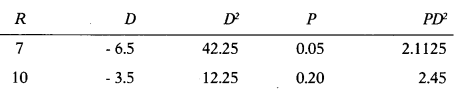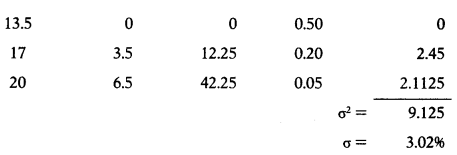Question 85.
Following details are available for EX Ltd. & FX Ltd. securities.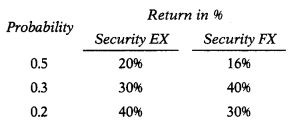Covariance between Security EX and Security FX is –
(A) 85
(B) 58
(C) -58
(D) -54
(B) 58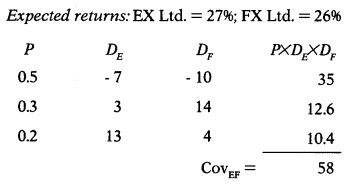Question 86.
Covariance between Security X and Security Y is + 45.8. This indicate that –
(A) If return on Security X is below par,return on Security Y is likely to be above par.
(B) Returns on Security X & Security Y tend to go together, i.e. if return on X is above par, return on Y is also likely to be above par.
(C) Returns on Security X & Security Y bear a tendency to off-set each other.
(D) Covariance of these to two securities is positive which means standard deviation of both securities must be negative.
(B) Returns on Security X & Security Y tend to go together, i.e. if return on X is above par, return on Y is also likely to be above par.

Question 87.
Covariance between Security X and security Y is – 42. This indicate that –
(A) Returns on Security X & Security Y bear a tendency to off-set each other.
(B) Covariance of these two securities is negative that means standard deviation of both securities must be positive.
(C) There is no distinct relation between Security X and Security Y.
(D) Return on Security X is above par, return on Security Y is also likely to be above par.
(A) Returns on Security X & Security Y bear a tendency to off-set each other.

Question 88.
Covariance between Security P & Q is 48.91. Standard deviation of Security P is 5.36 while that of Security Q is 9.13. Compute value of correlation coefficient.
(A) 0
(B) 1
(C) -1
(D) 0.899
(B) 1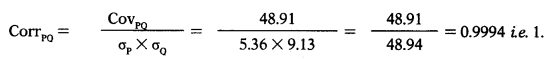Question 89.
An investor has invested in Security A & B in the ratio of 70:30. Standard deviation of Security A & B is 4.47 & 7.62 respectively. Covariance AB is 34. Correlation Coefficient of Security A & B is 1. Risk of portfolio is –
(A) 5.41%
(B) 6.48%
(C) 3.13%
(D) 7.26%
(A) 5.41%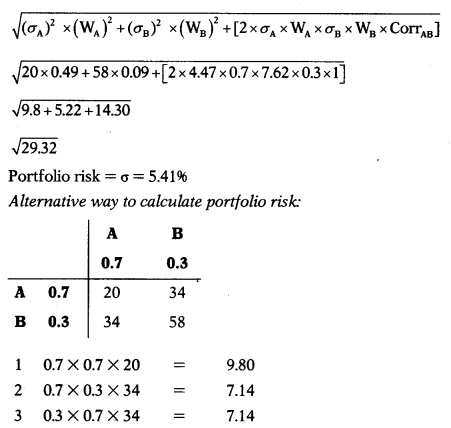Question 90.
X and Y are two securities in portfolio for which available. following information is …………..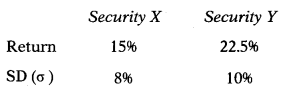Correlation coefficient is + 0.7.
What weight you will assign to the Security Y so that portfolio risk is lowest.
(A) 15.38%
(B) 84.62%
(C) 82.46%
(D) 20.18%
(B) 84.62%Question 91.
Suppose risk free rate is 4% and λ is 2.5%. If an investor takes 13% risk, he can expect a return of –
(A) 32.5%
(B) 52%
(C) 10%
(D) 36.5%
(D) 36.5%
Risk free rate is 4% and λ is 2.5%. Thus, for every 1% increase in the risk, the investor can expect 2.5% above risk-free return. Thus, if an investor takes 13% risk, he can expect a return of 36.5%
(13 × 2.5 + 4).

Question 92.
Yogesh invest ₹ 1,25,000 in shares of BABA Ltd., a listed company. At the end of period investment value is ₹ 1,32,000.He gets dividend of ₹ 8,000. Return from investment is –
(A) 11%
(B) 12%
(C) 13%
(D) 14%
(B) 12%
[(1,32,000 – 1,25,000) + 8,000]/1,25,000 12%

Question 93.
Following data is available for two listed securities:
Covrb = 37
σb = 8.49%
Correlation coefficient between security R and Security B is +0.8595. The standard deviation of Security R should have been -………..
(A) 4.03%
(B) 8.04%
(C) 5.07%
(D) 6.09%
(C) 5.07%
Covariance = Corrrb × σr × σb
37 = 0.8595 × σr × 8.49
37 = 7.297 × σr
σ = 5.07

Question 94.
Actual return of GK Ltd. for last four year is 20%, 14%, 17% and 18%. GLtd. has beta of 1.15. Return on market portfolio is 15%. Risk free rate of return is 6%. Compute Alpha value and decide whether to hold, buy or to sell the security.
(A) Alpha value is + 0.8 and hence it is advised to sell the security.
(B) Alpha value is – 0.9 and hence it is advised to buy the security.
(C) Alpha value is – 0.8 and hence it is advised to short sell the security
(D) Alpha value is + 0.9 and hence it is advised to buy the security.
(D) Alpha value is +0.9 and hence it is advised to buy the security.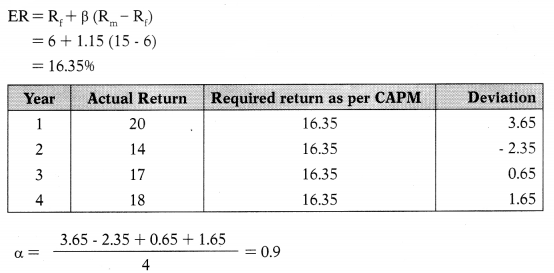Question 95.
Return of last 5 years of listed security is 16.2%, 19.8%, 18%, 15% & 21%. Five years ago price of the security was 120 per share. What is its average return
(A) 18%
(B) 19%
(C) 20%
(D) 21%
(A) 18%

Question 96.
Return of last 5 years of listed security is 16.2%, 19.8%, 18%, 15% & 21%. Five years ago price of the security was 120 per share. What is its holding period return
(A) 135.55%
(B) 128.58%
(C) 145.64%
(D) 154.45%
(B) 128.58%
120 × 1.162 × 1.198 × 1.18 × 1.15 × 1.21
= 274.29 (274.29 – 120)/120 × 100 = 128.58%

Question 97.
Dividend for last 4 years of Tara Ltd. was ₹ 7, ₹ 5,12.8 & ₹ 10 and market price was ₹ 120, ₹ 80, ₹ 130 & ₹ 150 respectively. What is the average return of last 3 years considering capital gain and dividend.
(A) 19.28%
(B) 14.48%
(C) 10.84%
(D) 16.65%
(A) 19.28%Question 98.
Given the probability of 0.1, 0.4, 0.3 & 0.2 estimated returns of the security are 36%, 26%, 20% & 15% respectively. Risk free rate is 6% and cost of capital is 13%. Total risk of security is –
(A) 36%
(B) 12%
(C) 6%
(D) 4%
(C) 6%Question 99.
From the following data, you are required to calculate the covariance of Security G and Security K and interpret the result.Select the correct answer from the options given below.
(A) Covariance between Security G & Security K is+56 whichindicate that return on these two securities tend to go together.
(B) Covariance between Security G & Security K is – 48 which indicate that return on these two securities bear tendency to off-set each other.
(C) Covariance between Security G & Security K is + 48 which indicate that return on these two securities tends to go together.
(D) Covariance between Security G & Security K is -56 which indicate that return on these two securities bear tendency to off-set each other
(D) Covariance between Security G & Security K is -56 which indicate that return on these two securities bear tendency to off-set each other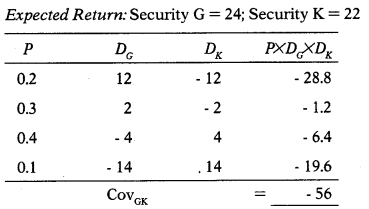Question 100.
Following data is available for the Security P & Q.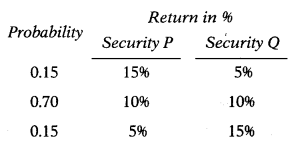Correlation Coefficient for the above two securities is –
(A) – 0.9899
(B) – 0.9989
(C) + 0.8999
(D) + 0.9989
(B) – 0.9989
Expected return of both securities is 10% and both securities have same probabilities for the returns hence standard deviation for both the securities will be same as shown below.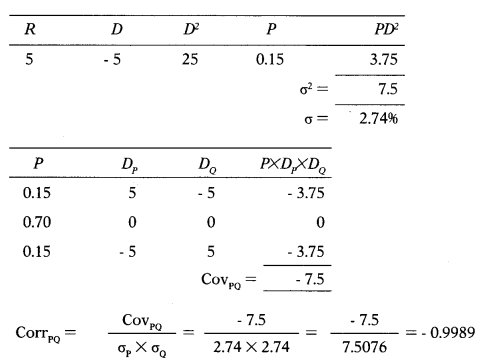Question 101.
Given the probability of 0.05,0.20,0.50, 0.20,0.05 estimated returns of Security A are 14%, 20%, 27%, 34% & 40% respectively. For same probability estimated returns of Security B are 10%, 20%, 28%, 36% & 46% respectively. Which security you will choose for investment and why.
(A) Total risk of Security A is 7.42% while that of Security B is 5.47% hence Security B will be selected.
(B) Covariance between Securities A & Security B is positive ie. +48 hence both securities can be selected.
(C) Expected return of Security B is 28% which is more than return of Security A which has return of 27% and hence Security B will be selected.
(D) Both securities have near about same returns ie.21% and 28% but standard deviation of Security A [6.04%] is less than standard deviation of Security B [7.62%] hence security A will be selected.
(D) Both securities have near about same returns ie.21% and 28% but standard deviation of Security A [6.04%] is less than standard deviation of Security B [7.62%] hence security A will be selected.
Security k (0.05 × 14) + (0.20 × 20) + (0.50 × 27) + (0.20 × 34) + (0.05 × 40) = 27%
Security B: (0.05 × 10) + (0.20 × 20) + (0.50 × 28) + (0.20 × 36) + (0.05 × 46) = 28%Question 102.
Raman has made investment in two securities in ratio of 60:40. Following data is available for these two securities:
Standard — Deviation:
Security —  A: 6.04%
Security —  B: 7.62%
Covariance between Security A & B is +45.80. Raman’s portfolio risk is –
(A) 6.66%
(B) 5.84%
(C) 3.67%
(D) 9.43%
(A) 6.66%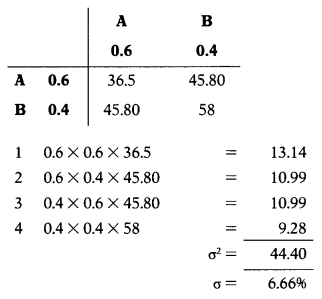Question 103.
Ram has formed portfolio with 3 securities. Proportion of investment in three securities is 35%, 25% & 40%.Ram’s portfolio risk = ₹
(A) 9.40%
(B) 3.41%
(C) 5.32%
(D) 4.76%
(B) 3.41%Question 104.
NSZ has formed portfolio with three securities P, Q & R. Proportion of investment in three securities is 20%, 70% & 10%. Other data of his portfolio is as follows:Risk of portfolio = ₹
(A) 9.90%
(B) 11.22%
(C) 10.45%
(D) 8.34%
(A) 9.90%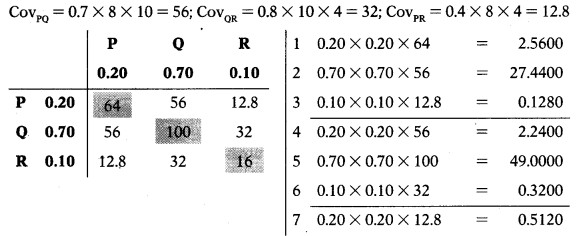Question 105.
K and H are two securities in portfolio for which following information is available.Correlation coefficient is + 0.5. Calculate the risk of portfolio using 20:80 weights.
(A) 12.13%
(B) 1321%
(C) 13.12%
(D) 12.31%
(C) 13.12%Question 106.
K and H are two securities in portfolio for which following information is available.Correlation coefficient is + 0.5. At what weight portfolio risk will be lowest.
(A) 87.51:12.49
(B) 58.71:41.29
(C) 84.17:15.83
(D) 14.29:85.71
(D) 14.29:85.71Question 107.
Security A has return of 12% with 10% risk (σ). Security B has return of 18% with 15% risk (σ). If investor makes investment in two securities in weight of 20:80 his return for portfolio is 16.80% with 13.12% risk (σ). If investor makes investment in two securities in weight of 14.29:85.71 his return for portfolio is 17.14% with 13.63% risk (σ). Which of the following strategy will be adopted by the rational investor
(A) Invest 100% in Security A.
(B) Invest 100% in Security B.
(C) Invest the ratio of 20:80 in A & B.
(D) Invest the ratio of 14.29 : Security A & B.
(D) Invest the ratio of 14.29 : Security A & B.In all the above strategy return is more than risk. Hence, depend upon risk tolerance investor can choose any strategy he likes. 1f investor is willing to take high risk, he wifi be get high return. At strategy (2) return is high Le. 18% but risk is also high. But as rational investor, it advised to choose strategy (4) as risk is lowest and return is also high as compared to risk.

Question 108.
Following is securities: the data regarding six securities: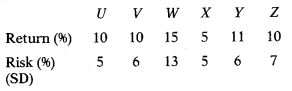Which three securities will be selected?
(A) Securities U, V & X
(B) Securities V, W & Z
(C) Securities U, W & Y
(D) Securities W, X & Y
(D) Securities W, X & Y
A rational investor will always choose those securities which have high return and low risk.
(1) Security U has return of 10% at a risk of 5%. Security V & Z also has returns of 10% but their risk is 6% & 7% which is more than risk of Security U and hence Security U dominates Security V & Z. Thus, out of Security U, V & Z, Security U can be selected.
(2) Security X has return of 5% and also has risk of 5%. Thus, investment in this security can be ignored for two reason – First, low return and secondly due same risk ie. risk is equal to return.
(3) In Security W & Y risk is high but return is also high.
In view of above observations, investment in Security U, W & Y can be selected based on individual prefer

Question 109.
Standard deviation of Market is 8.4%. Covariance of Security X with Market is 35.7. Risk free rate is 7% while GDP of the economy is growing at 8%. What is market sensitivity index of Security X?
(A) 1.315
(B) 0.879
(C) 0.506
(D) 0.605
(C) 0.506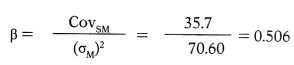Question 110.
Standard deviation of Security X and Market are 12% & 16% respectively. Covariance of Security X with Market is +96. What is market sensitivity index of Security X?
(A) 0.407
(B) 0.873
(C) 0.375
(D) 0.573
(C) 0.375Question 111.
Beta factor of Security Z is 0.506. Market risk is 8.4%. Correlation coefficient for Security Z and Market is 0.3965. What is the standard deviation of security Z?
(A) 11.45%
(B) 12.44%
(C) 10.98%
(D) 10.72%
(D) 10.72%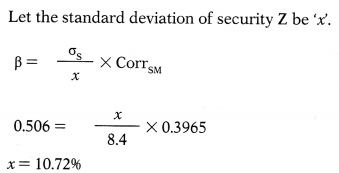Question 112.
Beta factor of Security Z is 0.506. Market risk is 8.4%. Correlation coefficient for Security Z and Market is 0.3965. Covariance between Security Z and x Market is -…………..
(A) 35.7
(B) 38.4
(C) 33.3
(D) 32.8
(A) 35.7Question 113.
Return on XM Ltd. shares has a standard deviation of 23%, as against the standard deviation of the market at 19%. Correlation co-efficient between market and stock of XM Ltd. is 0.8. Compute the systematic risk of XM Ltd.’s shares.
(A) 96.84%
(B) 18.4%
(C) 25.78%
(D) 64.85%
(B) 18.4%β = 0.9684
Systematic risk = Beta × SD of market
=0.9684 × 19
= 18.4%

Question 114.
Return on Lucky Ltd. shares has a standard deviation of 20%, as against the standard deviation of the market at 15%. Correlation co-efficient between market and stock of XM Ltd. is 0.9. Compute the unsystematic risk of Lucky Ltd.’s shares.
(A) 5
(B) 2%
(C) 18%
(D) 20%
(B) 2%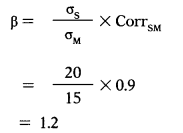Systematic risk Beta × SD of market
= 1.2 × 15
=18%
Unsystematic risk Total risk – Systematic risk
=20 – 18
=2%

Question 115.
You are analyzing the beta for ABC Ltd. and have divided the Company into four broad business groups, with market ; values and betas for each group.Estimate the beta for ABC Ltd. as a company.
(A) 1.275
(B) 1.825
(C) 1.645
(D) 0.895
(A) 1.275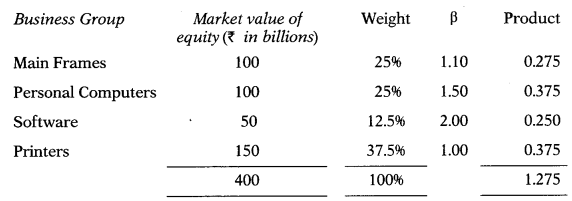Question 116.
Pavan has invested in four securities. Amount invested and beta of each security is as follows:Compute portfolio beta.
(A) 1.524
(B) 1.874
(C) 0.789
(D) 1.315
(D) 1.315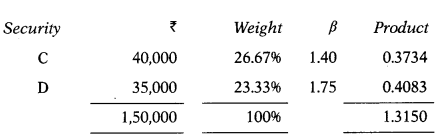Question 117.
ABC Ltd beta is 1.45. Rate of market return is 16%. Rate of return on government securities is 8%. What is the expected return as per Capital Asset Pricing Model? If the f risk premium on the market goes up by 2.5% points, what would be revised expected return on this stock?
(A) 16.9%; 32.32%
(B) 18.7%; 24.28%
(C) 19.6%; 23.23%
(D) 19.6%; 16.7%
(C) 19.6%; 23.23%
ER=Rf+p(Rm-Rf)
= 8 + 1.45 (16 – 8)
= 19.6%
Revised expected return if the risk premium on the market goes up by 2.5%:
ER = Rf + p (Rm – Rf)               Note: Risk Premium = (Rm – Rf)
= 8 + 1.45 (10.5)                     Revised risk premium = 8 + 2.5 = 10.5
= 23.23%

Question 118.
A financial consultant has gathered following facts for H Ltd.
Systematic risk of the firm is 1.4.
182 days Treasury bill yield is 8%
Expected yield on market portfolio is 13%. Calculate expected return based on capital asset pricing model (CAPM).
(A) 18%
(B) 17%
(C) 16%
(D) 15%
(D) 15%
ER= Rf+ p (Rm– Rf)
= 8+1.4(13 – 8)
= 15%

Question 119.
An investor is seeking the price to pay for a security, whose standard deviation is 3%. The correlation coefficient for the security with the market is 0.8 and the market standard deviation is 2.2%. The return from government security is 5.2% and from the market portfolio is 9.8%. The investor knows that, by calculating the required return, he can then determine the price to pay for security. What is the required return on the security
(A) 8.44%
(B) 12.66%
(C) 10.22%
(D) 9.77%
(C) 10.22%Question 120.
Calculate the required return on the security from the following information:
Standard deviation — 2.5%
Market standard deviation — 2.0%
Risk free rate of return — 13%
Expected rate of return on market portfolio 15%
Correlation coefficient of portfolio with the market 0.8
(A) 16%
(B) 15%
(C) 14%
(D) 12%
(B) 15%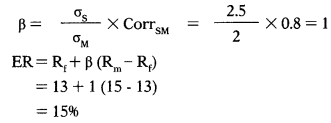Question 121.
As an Investment Manager you are given the following information: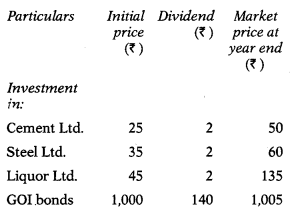Risk free return may be taken at 14%. Calculate expected rate of return on market portfolio.
(A) 26.33%
(B) 62.32%
(C) 24.78%
(D) 34.12%
(A) 26.33%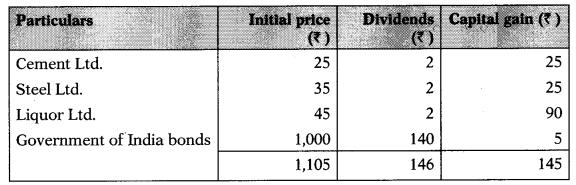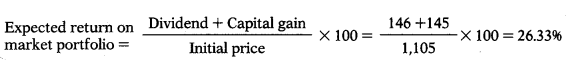Question 122.
As an Investment Manager you are given the following information:
Particulars — β
Cement Ltd. — 0.8
Steel Ltd. — 0.7
Liquor Ltd. — 0.5
GOl bonds — 0.99
Risk free return may be taken at — 14%.
Expected rate of return on market portfolio is — 26.33%.
Average return on portfolio = ?
(A) 23.86%
(B) 22.63%
(C) 26.21%
(D) 23.22%
(D) 23.22%
ER=Rf+ (Rm– Rf)
Cement Ltd. = 14 + 0.8 (26.33 – 14) 23.86%
Steel Ltd. = 14+0.7 (26.33 – 14) = 22.63%
Liquor Ltd.= 14+0.5 (26.33 – 14) = 20.17%
GOl Bonds 14+0.99(26.33- 14)26.21%Question 123.
A Ltd. has an expected return of 21% and standard deviation of 39%. B Ltd. has an expected return of 23% and standard deviation of 37%. A Ltd. has beta of 0.76 and B Ltd. a beta of 1.14. Market return is 20%. A rational and risk averse investor shall make investment in –
(A) A Ltd. as its standard deviation is greater than B Ltd.
(B) A Ltd. as greater risk means possibility of high returns.
(C) A Ltd. as its beta is less than B Ltd.
(D) B Ltd. as it expected return is more than A Ltd. and its risk is also as compared to A Ltd.
(D) B Ltd. as it expected return is more than A Ltd. and its risk is also as compared to A Ltd.

Question 124.
A Ltd. has an expected return of 22% and standard deviation of 40%. B Ltd. has an expected return of 24% and standard deviation of 38%. A Ltd. has beta of 0.86 and B Ltd. a beta of 1.24. The correlation of coefficient between return of A Ltd. and B Ltd. is 0.72. The standard deviation of market return is 20%. If you invest 30% in B Ltd. and 70% in A Ltd. what is your expected return and portfolio standard deviation?
(A) 22.6%; 35.74%
(B) 24%; 35%
(C) 22.6%; 37.06%
(D) 22.6%; 34.75%
(C) 22.6%; 37.06%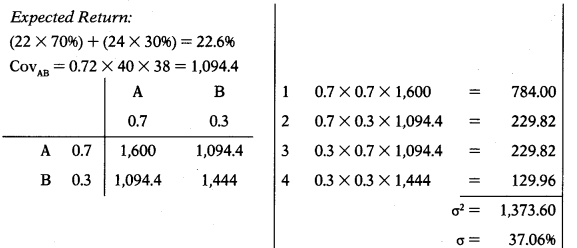Question 125.
Expected return of Security A is 22% while that of Security B is 24%. Beta of Security A is 0.86 while that of Security B is 1.24. What is risk free rate?
(A) 14.74%
(B) 17.47%
(C) 14.71%
(D) 14.00%
(B) 17.47%22= x+0.86y
24 = x+ 1.24y
– 2= – 0.38y
y = 2/0.38 = 52632
y = Rm – Rf = 5.2632
Putting value of ‘y’ in first equation we can get value of ‘x’.
22=x +0.86y
22 = x + 0.86 × 5.2632
22x + 4.5264
x = Risk free rate = 17.47%

Question 126.
Expected return of Security A is 20% while that of Security B is 25%. Beta of Security A is 0.85 while that of Security B is 1.25.
What is market rate of return?
(A) 21.875%
(B) 22.385%
(C) 18.655%
(D) 20.555%
(A) 21.875%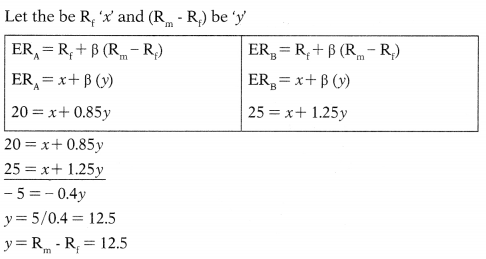Putting value of y in first equation we can get value of x.
22 = x+ 0.85y
22 = x+ 0.85 × 12.5
20 = x+ 10.625
20 = x+ 4.5264 x = Risk free rate = 17.47%

Question 127.
The expected return and beta of three securities is as follows:If the risk free rate of return is 6.75% and the expected return on market portfolio is 10.5%, which of the above securities under or correctly valued?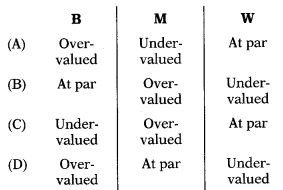(C)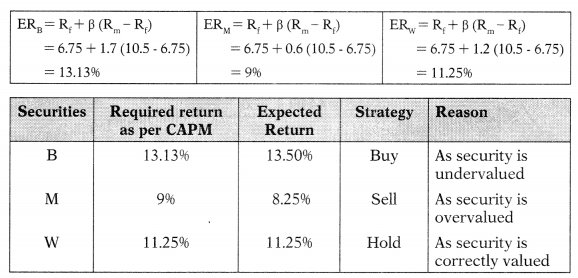Question 128.
Actual return of T Ltd. for last four year is as follows:
Year — Return
1 — 15.06%
2 — 10.12%
3 — 12.28%
4 — 13.26%
T Ltd. has beta of 1.15. Return on market portfolio is 11.2%. Risk free rate of return is 4%. Compute Alpha value and decide whether to hold, buy or to sell the security.
(A) Alpha value is positive Le. 0.4 and hence security is overvalued. Such security should be sold.
(B) Alpha value is positive Le. 0.4 and hence security is undervalued. Such security should be bought.
(C) Alpha value is negative Le. – 0.2 and hence security is overvalued. Such security should be sold.
(D) Alpha value is negative Le. – 0.2 and hence security is undervalued. Such security should be bought.
(B) Alpha value is positive Le. 0.4 and hence security is undervalued. Such security should be bought.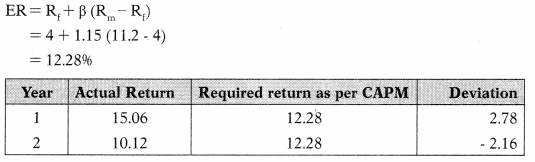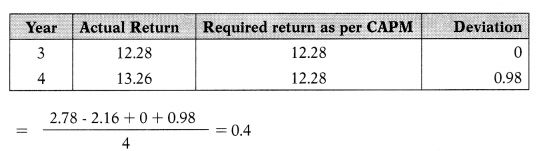Alpha value is positive ie. 0.4 and hence security is undervalued. Such security should be bought.

Question 129.
Following details are made available to you for a security.
Beta (β) : 1.4
Risk free rate of return : 6.3%
Market rate of return : 13.2%
If the alpha value is + 1.8 what investment action would you suggest?
(B) Hold
(C) Sell
(D) Short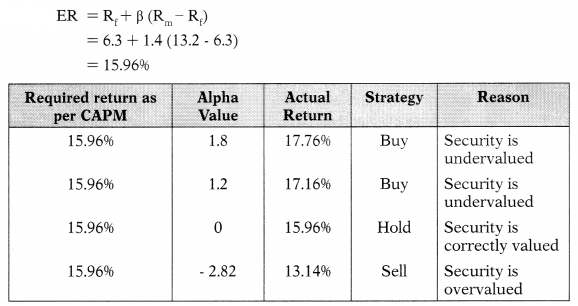Question 130.
Following details Eire made available to you for a security.
Beta (β) : 1.4
Risk free rate of return : 6.3%
Market rate of return : 13.2%
If the alpha value is + 1.2 what investment action would you suggest?
(A) Short
(B) Sell
(D) HoldQuestion 131.
Following details are made avaiable to you for a security.
Beta (β) : 1.4
Risk free rate of return : 6.3%
Market rate of return : 13.2%
If the alpha value is zero what investment action would you suggest?
(A) Buy as Security is undervalued.
(B) Sell as Security is undervalued.
(C) Hold as Security is correctly valued.
(D) Sell as Security is overvalued.
(C) Hold as Security is correctly valued.Question 132.
Following details are made avaUable to you for a security.
Beta (β) : 1.4
Risk free rate of return : 6.3%
Market rate of return : 13.2%
If the alpha value is negative i..e. – 2.82 what investment action would you suggest?
(A) Hold
(C) Sell
(D) None of the above
(C) SellQuestion 133.
Stocks A & B have the following historical returns: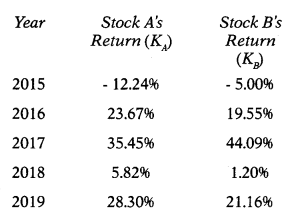Assume that someone held a portfolio consisting of 70% of Stock A and 30% Stock B. What would have been the realized rate of return on the portfolio in each year from 2015 to 2019?
(A) 16.20%
(B) 15.40%
(C) 18.60%
(D) 13.70%
(A) 16.20%Question 134.
Following details are gathered by the investor:
Standard deviation — 2.8%
Market standard deviation — 2.3%
Risk free rate of return — 8%
Expected rate of return on market portfolio — 18%
Correlation coefficient of portfolio with the market — 0.8
Required return on security is –
(A) 14.77%
(B) 17.74%
(C) 15.84%
(D) 20.65%
(B) 17.74%Calculation of required return on the security:
ER=Rf+β(Rm-Rf)
= 8 + 0.974(18 – 8)
= 17.74%

Question 135.
to you for particular security:
Beta of security : 0.5
Expected return on portfolio : 15%
Risk free rate of return : 0.06
In another security has an expected rate of return of 18%, what would be its beta?
(A) 1.222
(B) 1.111
(C) 1.333
(D) 1.444
(C) 1.333
ER= Rf+ P (Rm– Rf)
= 6 + 0.5(15-6)
= 10.5%
Computation of beta for other security:
ER=Rf+β(Rm-Rf)
18 = 6 + β (15 – 6)
18 = 6 + 9β
12 = 9β
P = 1.3333

Question 136.
Following information is available in respect of the return from Reliance’s stock under different economic conditions:Find out risk associated with it
(A) 5.6%
(B) 6.5%
(C) 8.4%
(D) 4.8%
(A) 5.6%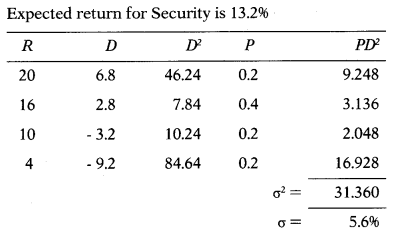Question 137.
The market portfolio has a historically based expected return of 10% and a standard deviation of 4% during a period when risk-free assets yielded 3%. The 7% risk premium is thought to be constant through time. You are required to find market’s return-risk trade-off.
(A) 1.25
(B) 1.50
(C) 1.75
(D) 2.00
(C) 1.75Question 138.
The market portfolio has a historically based expected return of 0.10 and a standard deviation of 0.04 during a period when risk-free assets yielded 0.03. The 0.07 risk premium is thought to be constant through time. Riskless investments may now be purchased to yield 0.09. A security
has a standard deviation of 0.08 and a coefficient of correlation with the market portfolio is 0.85. The market portfolio is now expected to have a standard deviation of 0.04. You are required to find equilibrium required expected return of the security.
(A) 11.4%
(B) 13.6%
(C) 18.9%
(D) 20.9%
(D) 20.9%Question 139.
Dhanpat, an investor, is seeking the price to pay for a security, whose standard deviation is 5%. The correlation coefficient for the security with the market is 0.75 and the market standard deviation is 4%. The return from risk-free securities is 6% and from the market portfolio is 11%. Dhanpat knows that only by calculating the required rate of return, he can determine the price to pay for the security. What is the required rate of return on the security?
(A) 10.69%
(B) 16.90%
(C) 19.60%
(D) 16.96%
(A) 10.69%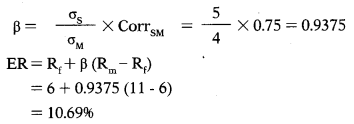Question 140.
Security-A offers an expected rate of return of 14% with a standard deviation of 8%. Security-B offers an expected rate of return of 11% with a standard deviation of 6%. If an investor wishes to construct a portfolio with a 12.8% expected return, what percentage of the portfolio will consist of Security-A & Security-B?
(A) 40:60
(B) 60:40
(C) 70:30
(D) 30:70
(B) 60:40
Let the weight of investment in Security-A be ‘x\ Thus, investment in Security-B = [1 – x\ Expected Return = (RA × WA) + (RB × WB)
12.8 = (14 × x) + (11 × [1 – x])
12.8 = 14x+ 11- 11x
1.8 = 3x
x = 0.6
Thus,
Weight of Security-A = 60%
Weight of Security-B = 40% (100- 60)

Question 141.
Mohan has a portfolio of 6 securities, each with a market value of Rs. 10,000. The current beta (β) of the portfolio is 1.30 and β of the riskiest security is 1.80, Mohan wishes to reduce his portfolio β to 1.15 by selling the riskiest security and replacing it with another security with a lower β. What must be the β of the replacement security?
(A) 0.8
(B) 0.7
(C) 0.9
(D) 1.1
(C) 0.9
Beta of a portfolio = Weighted average beta of the securities = ∑WB
Current beta of Mohan’s portfolio = 1.30
Market value of total portfolio = 10,000 × 6 = 60,000
Weight of each security in the portfolio = $$\frac{10,000}{60,000}=\frac{1}{6}$$
Sum of betas of six securities = 1.30× 6 = 7.80
Beta of portfolio after replacement of security =1.15
Sum of betas of six securities after replacement =1.15×6 = 6.90
Since the riskiest security with beta of 1.80 is to be replaced
Sum of betas of six securities – Beta of security to be replaced = Sum of betas of six securities after replacement
7.80- 1.80 + x = 6.90
x = 0.90
Thus, beta of security replaced should be 0.90.

Question 142.
Zebra Ltd. has a beta (β) of 1.15. The return on market portf oho is 14%. The risk free rate of return is 5%. Actual rates of returns over 4 observations are as under:(A) Year 1 & Year 3
(B) Year 2 & Year 3
(C) Year 3 & Year 4
(D) Year 1 & Year 4
(D) Year 1 & Year 4
ER = Rf + β (Rm – Rf)
= 5 + 1.15 (14 – 5)
= 15.35%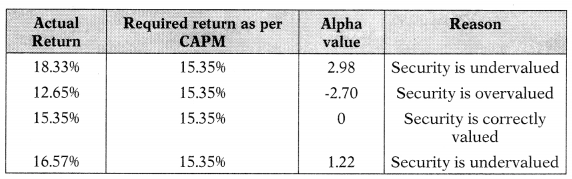Question 143.
Return on Lucky Ltd.’s shares has a standard deviation of 22%, as against the standard deviation of the market at 12%. Correlation co-efficient between market and stock of Lucky Ltd. is 0.7%. Compute unsystematic risk of Lucky Ltd.’s shares.
(A) 15.4%
(B) 8.4%
(C) 6.6%
(D) 12.6%
(C) 6.6%$$\beta=\frac{22}{12} \times 0.7$$
β = 1.2833
Systematic risk = Beta × SD of market
= β × σM
= 1.2833 ×12
= 15.4%
Unsystematic risk = Total risk – Systematic risk
= 22 – 15.4
= 6.6%

Question 144.
In a portfolio of the company, ₹ 2,00,000 have been invested in Asset-X which has an expected return of 8.5%, ₹ 2,80,000 in Asset-Y, which has an expected return of 10.2% and ₹ 3,20,000 in Asset-Z which has an expected return of 12%. What is the expected return for the portfolio?
(A) 12.945%
(B) 10.495%
(C) 10.549%
(D) 15.945%
(B) 10.495%Question 145.
The following data relate to two securities, A and B: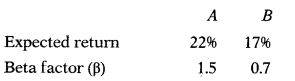Assume RF 10% and RM = 18%.
Find out whether the securities, A and B are correctly priced?
Security A — Security B
(A) Undervalued — Overvalued
(B) Overvalued — Undervalued
(C) Correctly valued — Under valued
(D) Overvalued — Correctly valued